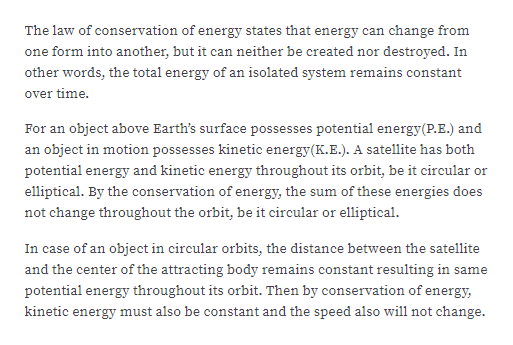# Is the sum of kinetic and potential energies a constant for satellites in circular orbits, in elliptical orbit or in both?

Question
1 views

Is the sum of kinetic and potential energies a constant for satellites in circular orbits, in elliptical orbit or in both?

check_circle

Step 1

Determining if the sum of potential energy and kinetic...help_outlineImage TranscriptioncloseThe law of conservation of energy states that energy can change from one form into another, but it can neither be created nor destroyed. In other words, the total energy of an isolated system remains constant over time. For an object above Earth's surface possesses potential energy(P.E.) and an object in motion possesses kinetic energy(K.E.). A satellite has both potential energy and kinetic energy throughout its orbit, be it circular or elliptical. By the conservation of energy, the sum of these energies does not change throughout the orbit, be it circular or elliptical. In case of an object in circular orbits, the distance between the satellite and the center of the attracting body remains constant resulting in same potential energy throughout its orbit. Then by conservation of energy, kinetic energy must also be constant and the speed also will not change. fullscreen

### Want to see the full answer?

See Solution

#### Want to see this answer and more?

Solutions are written by subject experts who are available 24/7. Questions are typically answered within 1 hour.*

See Solution
*Response times may vary by subject and question.
Tagged in

### Gravitation